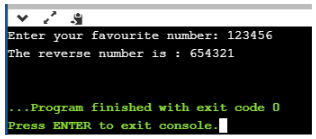# How to reverse a number in Python?7 / 11 Blog from Python Programs

Python is an interpreted, high-level, general-purpose programming language with different applications. To learn the fundamental concepts of Python, there are some standard programs which would give you a brief understanding of all the concepts practically. Reverse a number in Python is one of these programs which gives the learner a deep understanding of loops and arithmetic operators. This blog will help you understand and implement the ways to reverse a number. It will cover the following topics –

## How to reverse a number in Python?

It’s simple! You can write a Python program which takes input number and reverse the same. The value of an integer is stored in a variable which is checked using a condition and then each digit of the number is stored in another variable, which will print the reversed number. Numbers can be reversed in Python using different methods, let us take a look at the Python program to implement the same.

## Python program to reverse a number

There are two ways to reverse a number in Python programming language

• Using a Loop
• Using Recursion

## Reverse a Number using Loop

``` # Get the number from user manually
num = int(input("Enter your favourite number: "))

# Initiate value to null
test_num = 0

# Check using while loop

while(num>0):
#Logic
remainder = num % 10
test_num = (test_num * 10) + remainder
num = num//10

# Display the result
print("The reverse number is : {}".format(test_num))

```

Output:Program Explanation

User value: Number = 123456 and Reverse = 0

First Iteration
Reminder = Number %10
Reminder = 123456%10 = 6
Reverse = Reverse *10 + Reminder
Reverse = 0 * 10 + 6 = 0 + 6 = 6
Number = Number //10
Number = 123456 //10 = 12345

Second Iteration
From the first Iteration the values of both Number and Reverse have been changed as: Number = 12345 and Reverse = 6
Reminder = Number % 10
Reminder = 12345 % 10 = 5
Reverse = Reverse *10+ Reminder = 6 * 10 + 5
Reverse = 60 + 5 = 65
Number = Number //10 = 12345 //10
Number = 1234

Third Iteration
From the Second Iteration, the values of both Number and Reverse have been changed as: Number = 1234 and Reverse = 65
Reminder = Number %10
Reminder = 1234%10 = 4
Reverse = Reverse *10+ Reminder = 65 * 10 + 4
Reverse = 650 + 4 = 654
Number = Number //10 = 1234//10
Number = 123

Fourth Iteration
From the Second Iteration the values of both Number and Reverse have been changed as: Number = 123 and Reverse = 654
Reminder = Number %10
Reminder = 123 %10 = 3
Reverse = Reverse *10+ Reminder = 654 * 10 + 3
Reverse = 6540 + 3 = 6543
Number = Number //10 = 123//10
Number = 12

Fifth iteration
From the Second Iteration the values of both Number and Reverse have been changed as: Number = 12 and Reverse = 6543
Reminder = Number %10
Reminder = 12 %10 = 2
Reverse = Reverse *10+ Reminder = 6543 * 10 + 2
Reverse = 65430 + 2 = 65432
Number = Number //10 = 12//10
Number = 1

Sixth iteration
From the Second Iteration, the values of both Number and Reverse have been changed as, Number = 1 and Reverse = 65432
Reminder = Number %10
Reminder = 1 %10 = 1
Reverse = Reverse *10+ Reminder = 65432 * 10 + 1
Reverse = 654320 + 1 = 654321
Number ended:

## Reverse a Number using Recursion

```
# Python Program to Reverse a Number using Recursion

Num = int(input("Please Enter any Number: "))

Result = 0
def Result_Int(Num):
global Result
if(Num > 0):
Reminder = Num %10
Result = (Result *10) + Reminder
Result_Int(Num //10)
return Result

Result = Result_Int(Num)
print("n Reverse of entered number is = %d" %Result)
```

Output:With this, we come to an end of this blog on “Reverse a Number in Python”. I hope it added value to your knowledge of Python programming.

To get in-depth knowledge on Python along with its various applications, you can enroll here for live online training with 24/7 support and lifetime access. Got a question for us? Mention them in the comments section of “Reverse a Number in Python” and we will get back to you.

Upcoming Batches For Python Certification Training Course
Course NameDate
Python Certification Training Course

Class Starts on 21st October,2023

21st October

SAT&SUN (Weekend Batch)
View DetailsREGISTER FOR FREE WEBINARThank you for registering Join Edureka Meetup community for 100+ Free Webinars each month